# HSPT Math : Concepts

## Example Questions

1 2 146 147 148 149 150 151 152 154 Next →

### Example Question #2125 : Hspt Mathematics

A sphere has diameter 12. What is 75% of its surface area?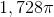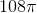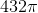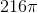Explanation:

The radius of a sphere is half its diameter, which here is 12, so the radius is 6. The surface area of the sphere can be calculated by setting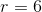in the formula: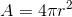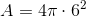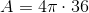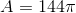75% of this is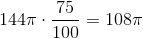### Example Question #2126 : Hspt Mathematics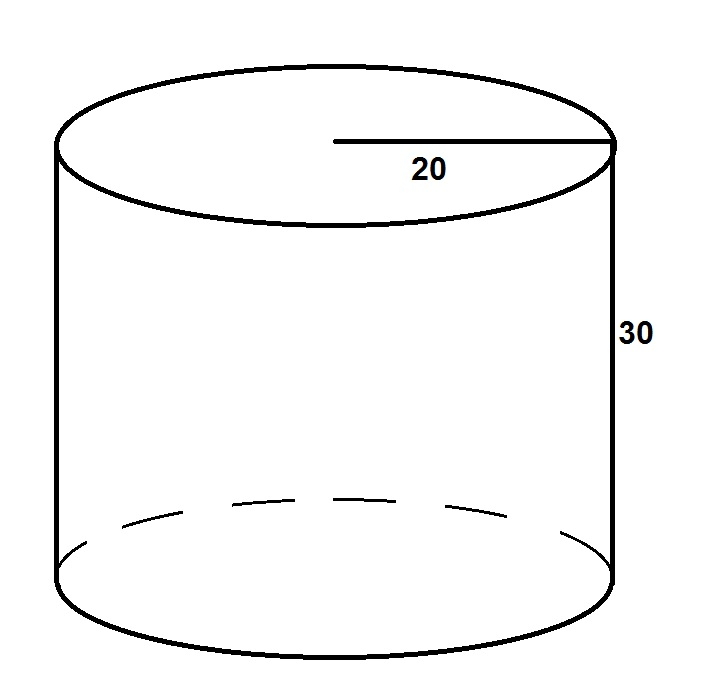Which expression is equal to 40% of the surface area of the above cylinder?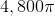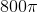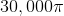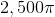Explanation:

The surface area of the cylinder can be calculated by setting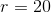and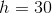in the formula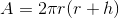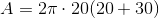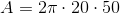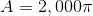40% of this surface area is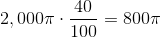1 2 146 147 148 149 150 151 152 154 Next →

### All HSPT Math Resources